# wiener法图像处理

## 维纳滤波器(Wiener Filter)在图像处理中的应用（一）

2015-03-22 20:39:49 ispforfun 阅读数 11809

维纳滤波器是一种自适应的滤波器，在数字信号处理中有着广泛的应用。ispforfun会在从今天开始定期给大家带来维纳滤波器在图像处理中应用。本节讲诉维纳滤波器在图像去噪中的简单应用。

让我们从Matlab中的函数wiener2开始。Matlab的help中对wiener2的说明如下：

wiener2估计图像中每个像素的局部均值和局部方差，

其中，是图像A中每个像素的NXM邻域。维纳滤波器的去噪原理如下：

其中是噪声的方差，如果没有给出来，那么会根据图像的局部方差进行估计。

ispforfun根据这段原理，编写了如下的matlab代码，共享给大家。

function J = wiener_image_denoising_filter(I, Win, noise_var)
% J = wiener_image_denoising_filter(I, w)
% -----------------------------------------
% by: ispforfun
% I is the input image. Single channel image.
% Win is the window size for filtering.

[img_height, img_width] = size(I);
half_win_size = floor(Win/2.0);

[X, Y] = meshgrid(1:img_width, 1:img_height);
[dX, dY] = meshgrid(-half_win_size(2):half_win_size(2), -half_win_size(1):half_win_size(1));

dX = reshape(dX, [1 1 Win(1) Win(2)]);
dY = reshape(dY, [1 1 Win(1) Win(2)]);
X = repmat(X, [1 1 Win(1) Win(2)]) + repmat(dX, [img_height img_width 1 1]);
Y = repmat(Y, [1 1 Win(1) Win(2)]) + repmat(dY, [img_height img_width 1 1]);

X(X<1) = 2 - X(X<1);
X(X>img_width) = 2*img_width - X(X>img_width);
Y(Y<1) = 2 - Y(Y<1);
Y(Y>img_height) = 2*img_height - Y(Y>img_height);

patch = @(f)f(Y + (X-1)*img_height);

P = patch(I);

img_mean = local_image_mean(P);
img_var = local_image_var(P, img_mean, Win);

if nargin < 3
estimated_noise_var = sum(sum(img_var))/(img_height * img_width);
else
estimated_noise_var = noise_var;
end

gain = (estimated_noise_var == 0.0).*zeros(img_height, img_width) + ...
(estimated_noise_var ~= 0.0).*((img_var - estimated_noise_var)./img_var);

J = img_mean + gain.*(double(I) - img_mean);

J = min(max(J, 0), 255);

J = uint8(J);

%gain = (img_var - estimated_noise_var)./img_var;

function img_mean = local_image_mean(P)
% img_mean = local_image_mean(P)

local_img_sum = sum(sum(double(P), 4), 3);
sum_size = size(P,3)*size(P,4);

img_mean = local_img_sum/sum_size;

function img_var = local_image_var(P, img_mean, Win)
% img_var = local_img_var(P, img_mean)

diff_img_patch = double(P) - repmat(img_mean, [1 1 Win(1) Win(2)]);
diff_img_patch_pow2 = diff_img_patch.^2;
local_diff_img_sum = sum(sum(double(diff_img_patch_pow2), 4), 3);
img_var = local_diff_img_sum/(Win(1)*Win(2) - 1);wiener法图像处理 相关内容

## 图像处理的常用方法

2015-09-24 13:36:09 Real_Myth 阅读数 6902

# 盲源分离：

## 色调以及摄影的应用

1. LCH，lab 只是不同的颜色模型，并且常见光线与颜色模型值，例如强度与功率等。
2. Recovering highlights with dcraw using LCH blending
3. 摄影用光 色温 色温中，绿色基本保持不变34％，我们通常指的色温调整主要改变是RB两个的百分比。三者靠近比例接近的时候就是白光也就是日光色温，日光的色温基本是5500k. 黑体色温与CIE的色温定义是有区别的。并且色温也是表征能量，而不是颜色的。不能表达所有颜色。
4. brucelindbloom 关于颜色各种转换与在线工具
5. Decoding raw digital photos in Linux
6. 色彩与色调的调整 要慢慢来看懂这些理论了与实际的用途了
7. 白平衡与直方图均衡化 这个到底是什么关系呢，是不是对应关系
8. 直方图、对比度调节及白平衡 可以做一下这些实验在，GIMP或者python中
9. 调色教程入门二——色彩基础,想学调色的必看 要看懂直方图的分布意义了
10. 色温表

## 思考

Hue-Saturation

#. Y U V introduction – Main.GangweiLi - 12 Aug 2012

– Main.GangweiLi - 20 Nov 2012

– Main.GangweiLi - 02 Jan 2013

# 图像降噪

k-L 变换就是求数据的协方差矩阵的特征值和特征向量。

## 基于PCA的降噪，

PCA降噪方法和之前的降维差不多，对于降噪来说，就是获得信号子空间和噪声子空间，-》从噪声子空间中估计噪声方差-》remove 噪声子空间

## 小波变换

haar变换，就是利用上一个点对下一点进行相减，在信号不变的情况下，得到的信号就是稀疏的，稀疏之后然后在通过设置门限就可以把噪声抑制掉。

## 相干斑

1. 基于multi-looking processing， 但是这样会降低雷达的分辨力（好像是方位分辨率吧）
2. 基于小波变换的方法。
3. 基于ICA的方法。
4. 基于自适应滤波的方法。
5. k-L变换 清华
6. k-L变换，里面还有一些基本的主分量分析，小波变换等的数学推导
8. klt找到脸位置，matlab代码
9. scikit-learn: machine learning in Python 有空看一下
10. PCA降噪matlab代码
11. PCA降噪python 代码 怎样装上他们开发的modulate
12. 自适应变换小波降噪
13. 多分辨率curvelet transform
14. wavelet.py 图像小波降噪

# 直方图：

## introduction

matlab实现：histeq函数。

1. RGB转灰度图的几种算法 RGB转换为灰度图完全是我们心理的作用.
2. 50 RGB向灰度转换的原理 可以看一下OPenCV直方图均衡化 <http://blog.csdn.net/luomaojiang/article/details/8601321>_ 这里两个图看很形象直观。但是观点是做的，那就是均衡化不会每个灰度级上都具有相同的象素点数过程。原来每一个篮子的小球的数量是不会改变的，会改变的，只是让原来篮子的大小，随着之进行改变。直方图维基定义 这里给出的定义，扩展常用的亮度。也就是概率最大的亮度之间的差值。

# ImageMagick

## Introduction

imagemagick 是一个超强的库，并且指命令行接口异常的强大，基本上图片处理，画图功能它都已经提供了。可以说是一个cli版的GIMP。 同时它又像其他的linux工具一样，它的功能一种是在编译时通过各种宏来指定。另一种方式那就是采用配置文件。并且采用一定的搜索算法。最先开始在当前文件夹下.magick文件开始。从了一些正常的参数之外，还可以通过+define指定的那些隐性的参数。并且用identify 命令可以读取也可以添加各种的元数据到图片中 <http://www.exif.org/>_exiv2 同时还能够把这些元数据给strip掉，就像gcc的编译一样。每一个图片本身就是对象，我可以图像的统计信息放在元数据里，放在comments里，并且它支持一些通配符。简单的命令可以用convert,mogrify,display等，脚本可以使用conjure <http://imagemagick.sourceforge.net/http/www/conjure.html#msl>_ .%RED%而这个正是想要的一直准备去做的一件事之一。如何实现一个动态搜索接口。win7的文件浏览器功能就不错。自己是不是可以基于开源的文件浏览器改出来一个。%ENDCOLOR%其实imagemagick提供了一整套的命令行，包括管理显示排序方面关于图片的，问题自己如何利用这些。 #. 如何显示那文件属性 可以直接使用identify,convert来查看与编辑图片的属性。 #. best-free-digital-image-viewer.htm

## 图片的显示管理

ImageMagick提供import进行截屏操作。montage,display,animate批量化的显示，动画的显示与修改.同时进行格式的转化，动画frame的控制 insert/swap/delete/delay/loop，并且能够直接操作.gif中frame.修改顺序。并且还能折分与提取与合成pdf,还能用来破解pdf.。 做和种可视化对比的利用montage 是非常方便的。根据结果生成各种各样对比图片数据。 并且在XWindows中,display可以指定的显示在哪一个窗口，并且还可以设为哪一个窗口的背景，并且还能实时监控图片的变化，vimdot就是这样实现的。但是如指定缩放呢。还是使用montage的方法。

## 图形的变换

compare 比较做的还是比较智能的，直接支持单通道的比较，而不需要先分离然后再比较。并且对于各种特效的变换。例如watermark 添加以及签名以及边框的添加都是高度可定制化与批量化的。另一个那就是图片的隐藏，实现的原理是什么呢，直接进行图片叠加算法，还是只在后面又加了一帧。应该不是又加了不是这种，应该是算法叠加。Steganography composite -stegano 直接转化为噪声隐藏位图的低位。然后再恢复按照固定的函数算法再恢复出来。但是这种方法也会受到图片的裁减的破坏。 是不是可以直接把ASCII码当灰度或者unicode码当做灰度值，然后把文字变成图像，同时也研究一个那个小鸭子是如何实现的。

 hmm-kalman-particle-filtering <http://freemind.pluskid.org/machine-learning/hmm-kalman-particle-filtering/>_
-segment 这个只是从直方图进行分割。
-Solarize  曝晒算法 <http://courses.washington.edu/hypertxt/cgi-bin/book/pmontage/solarized.html>_ 模仿最初沥清制图的方法，采用V字型反转就可以了对于曲线或者直方图
-sharpen 虽然都是高斯，取高通，还是低通，就看你用哪一部分，你是用3sigma之内就是低通，3sigma之外就是高通了
-Swirl 旋涡效果
 -threshold -level -modulate
-tint 这其实也是图像分割的一种 最简单的应用，在过滤的时候会非常的方便 threashold用的最大值，level用的最小值，其实就是直方图的输出 -tint 是调整各个分量的多少 -modulate 用的是HSV模型，而前面两个用的是RGB模型
-unsharp 去除模糊，其实锐化的工具
-wave 要么横向，要么纵向，能不能径向
-virtual pixel 是不是利用多点来表示一点，具体还不太明白
roll 自身左右循环移位与上下循环移位
-append 就像字符串拼接一样，把图片接在一起 自动去改文件头 并且能够指定边框，背景
-average -fatten 把多张图，叠加起，点对点按照不同的规则，就像两张的加减一样
-affinite 二维的变换矩阵，但是一个问题，那就是坐标原点在哪里

## 画图

-annotate 添加注注释
-pointsize 字体大小
-stroke 线条颜色
-fill
-font
-draw color 50 50 point/replace/foodfill
^ rectangle
^ circle
^ text
^ Image in/over/out/atop
composite
 -compose 合成图片

-write 可以保存每一步的中间状态

display 命令的用法可以按照slide来播放

Imagemagick中图片大小转换全攻略

# 图像恢复 （降噪）

G=F*H+N 还是G=H(F+N) 还是只有N，还是只有H，处理方法都是不样的。主要需要考虑的因素有 #. N是不是可分离的 #. H 是不是可以逆的，一个是指数学上，一个是指实践的处理对象上。 #.

 只有 G=F+N 并且N在唯一空间的，或者N的频谱明显与原图的频谱有距离，这一种是最简单的，只需要简单的高通，低通，带通，在二维还有多带通。 例如常规的，中值，均值，最大值，最小值，另外掩码的尺寸也是重要的因素在处理效果上

x(0)=w(0)y,
augy=(yy)(1)y;

w(1) = aug_y*y

err=y(eye(10)yw(i));

## 维纳滤波

wiener filtering是相对于 inverse filtering 和smooth filtering 的一个折中，目标函数 [ŝ s]2 最小，最后得出信号的系数（相对于噪声的系数），也就是要知道信号和噪声的方差。但是实际中这个怎样给出？但是并不是wiener filtering 对所有的 退化图像和噪声都有用， 我想还是根据不同的模型，设计不同的方法，学会建模，并且利用数学方程求解。无非就是就极值的问题。

## 图像复原小结

1. Multi-dimensional image processing 里面有专门的各种滤波器
2. noise 1.2.1 python噪声包
3. scipy.signal.wiener scipy和numpy的参考文档
4. Wiener Filtering matlab 程序
5. Wiener Filtering推导
6. 简单的同态滤波(matlab)
7. 同态滤波(Homomorphic filtering)
8. 雨滴去除 来这做一下这样的效果。
9. Wiener Filtering 应该比较详细，看一下
10. 穿墙雷达 超宽带波藉由墙壁反弹的方式?及高性能的处理器而找到障碍物。可判读出在极短时间内的变化产生轻微的动作

# 图像分割

## 线检测

1. 计算图像的梯度并对其设置门限得到一幅二值图像。
2. 在参数平面内确定再细分。
3. 对像素高度集中的地方检验其累加器的单元的数目。
4. 检验选择的单元中像素之间的关系。

## 图像分割——分水岭算法

1. OpenCV-Python教程（9、使用霍夫变换检测直线） 检测中线段，试一下

## +图像分割之（二）Graph Cut（图割） <http://blog.csdn.net/zouxy09/article/details/8532111>_

E(L)=αR(L)+B(L)

Rp(1)=lnPr(Ip|"obj")
Rp(0)=lnPr(Ip|"bkg")

B(L)=p,qNB<p,q>δ(lp,lq)

## -+grab-cut < 图像分割之（三）从Graph Cut到Grab Cut>_

D(x)=i=1Kπigi(x;μ,Σi),i=1Kπi=1and0πi1

g(x;μ,Sigma)=1((2π)dSigma|)exp[12(xμ)TSigma(1)(xμ)]

V(α,z)=γ(m,n)C[anam]expβ||zmzn||2

# 图像拼接

Ip=IWWp=I,[WxpWyp]

1. 一种基于区域匹配的图像拼接算法
2. 图像拼接算法及实现 拼接算法主要两类一类是分域匹配，另一个基于特征提取
3. 图像拼接Image Mosaic 北京联高软件开发有限公司

# 图像处理## 思考

– Main.GangweiLi - 16 Jun 2012

# 平滑空间滤波器

## 空间滤波实现滤波 和目标分割 （基本原理：积分用来模糊图像，微分用来锐化图像）

Roberts算子： 检测边缘，对噪声敏感。（实际中使用什么检测特性还不清楚）

Prewitt算子： 采用局部平均，对噪声有一定的抑制作用。

Laplace 算子： 具有各项同性微分算子，具有旋转不变性 <http://www.narutoacm.com/archives/laplace-rotation-invariant/>_ :

Laplace(f)=2fx2+2fy2

## 频域滤波

1. fftw3-dev GPL 库，并且支持 ARM NEON，作为以后移植方便。并且[[http://gfourier.sourceforge.net/ fftw 可以直接用到GIMP GFourier]]

## Thinking

1. 斜坡面上，一阶微分一直不为0 ；二阶微分只有终点和起点不为0 1. 一阶微分产生较粗的边缘，二阶微分则细得多 1. 一阶微分处理一般对灰度阶梯有较强的响应；二阶微分处理细节有较强的响应

C:快盘graphicpaper1673-2944(2012)03-0022-05.pdf 基于DFT压缩。

python 中怎样plt.axes 怎样调节参数

– Main.GegeZhang - 27 Jul 2013

*对一维到二维一直推广，其实是假定了，图像线性时不变系统。所以先算行或者例都是没有关系。这是由于线性系统自身性质决定的。

wiener法图像处理 相关内容

## MATLAB图像处理

2018-06-18 21:42:30 qq_41174320 阅读数 6553

# 一、图像处理预备知识

## 2、图像处理常用方法

### 6）图像识别

Matlab是一个高级的矩阵/阵列语言，它包含控制语句、函数、数据结构、输入和输出和面向对象编程特点。用户可以在命令窗口中将输入语句与执行命令同步，也可以先编写好一个较大的复杂的应用程序（M文件）后再一起运行。

# 三、MATLAB图像处理代码

## 1、图像读取及保存

MATLAB的基本数据单位是矩阵，一幅图像可定义为一个二维函数f(x,y)，其中x和y是空间（平面）坐标，而在任何一对空间坐标(x,y)处的幅值f称为图像在该点处的强度或灰度。当x，y和灰度值f是有限的离散数值时，我们称该图像为数字图像。在MATLAB中，数字图像就是一个个矩阵。

## 1）图像读取

### 2）图像保存

imwrite(OutImg,'d:\photo\yn_ln.jpg'); %将OutImg矩阵表示的图像存储到电脑D盘photo文件夹中，并命名为yn_ln的.jpg格式的图像。

### 3）图像显示

subplot(行数，列数，区域索引);

## subplot(1,1,1);imshow(InImg); %将InImg图像文件显示到屏幕上指定位置

### 4）图像转换

I=rgb2gray(rgbImg)： 将彩色图像转换为灰度图像，rgbImg为彩色图像
bw=im2bw(Img,level)： 将灰度图像转换为二值图像，Img为灰度图像，level为0~1的灰度级阈值；## 1）数字图像灰度增强

J=imadjust(I,[low high], [bottom top],gamma): 对灰度图像进行直接灰度变换；I为增强前灰度图像，J为增强后的灰度图像，[low high]增强前灰度级范围，[bottom top]增强后灰度级范围

clear;
I=rgb2gray(InImg);
bw1=im2bw(I,0.8);
bw2=im2bw(J,0.8);
bw2=medfilt2(bw2);
subplot(1,2,1);imshow(bw1);
subplot(1,2,2);imshow(bw2);## 2）数字图像空域滤波

S=medfilt2(J): 对图像进行中值滤波

M=0;V=0.01;
J1=imnoise(I, 'gaussian',M,V);
S=medfilt2(J1);
subplot(1, 2, 1); imshow(J1);
subplot(1, 2, 2); imshow(S);## 3）数字图像频域滤波

F=fft2(I): 2D 傅里叶正变换

Pha=angle(F): 计算相位谱

R= abs(F): 计算功率谱
F1=real(F): 获得傅里叶变换实部分量
F2=imag(F): 获得傅里叶变换虚部分量
fftshift(F): 移动傅里叶频谱中心至零频率

I=ifft2(F): 2D 傅里叶逆变换

I=BWBand(21,31);
F=fft2(I);                        %傅里叶正变换
F=fftshift(F);
L=abs(F);
L= mat2gray(L);
I3=imrotate(BWBand(21, 31),30);
F3=fft2(I3);
F3=fftshift(F3);
L3=abs(F3);
L3= mat2gray(L3);
subplot(141); imshow(I);
title('原图');
subplot(142); imshow(I3);
title('旋转30度图');
subplot(143); imshow(L);
title('功率谱图');
subplot(144); imshow(L3);

title('旋转30度功率谱图');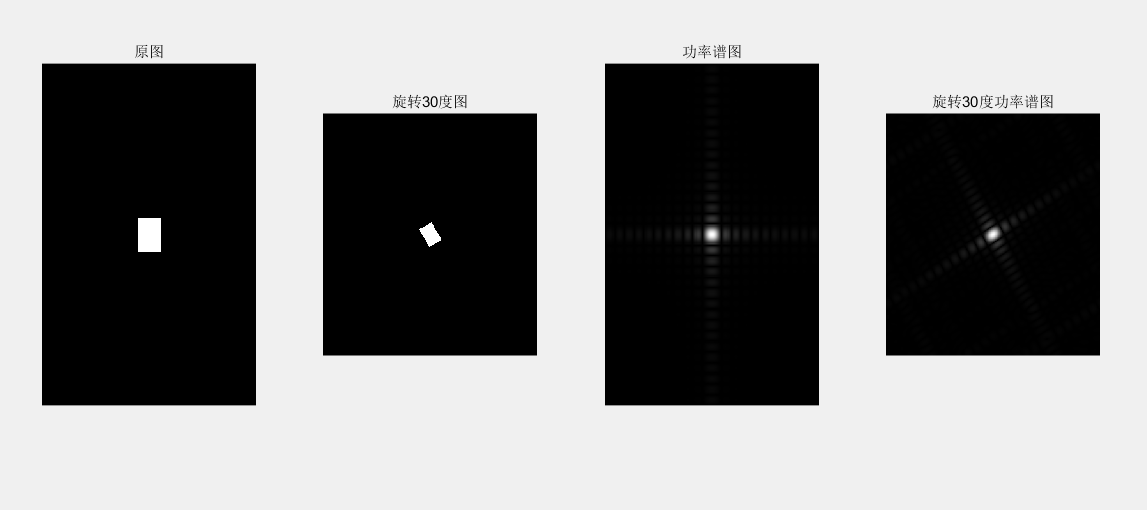## （2）InImg=imread('d:\photo\ying.jpg'); subplot(2, 2, 1); imshow(InImg);  title('原彩色图'); I=rgb2gray(InImg); J1=imnoise(I, 'salt & pepper',0.01); subplot(2, 2, 2); imshow(J1);  title('椒盐噪声污染图'); [f1, f2]=freqspace([199 200],'meshgrid');        %产生等间隔采样的频率响应 R=sqrt(f1.^2+ f2.^2);                   %计算功率谱 Hd=ones([199 200]); Hd(R>0.5)=0;                                   %产生理想的带通滤波器，频率带宽[0.1 0.5] win= fspecial('gaussian',[199 200],2); %采用高斯函数生成同样大小的窗函数 win= win./max(win(:));                       %窗口函数归一化 H=fwind2(Hd,win);                           %利用窗口函数产生实际的带通滤波器  subplot(2, 2, 3); freqz2(H); ff= imfilter (J1,H); subplot(2, 2, 4); imshow(ff);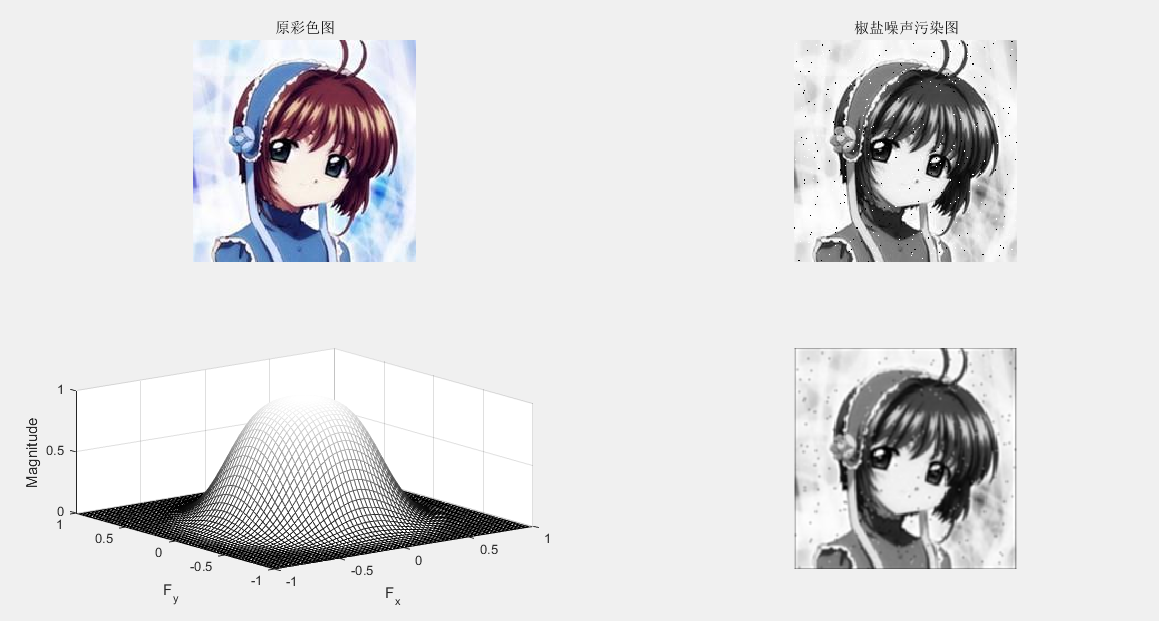## 3、图像复原

J = WIENER2(I,[M N]): 对灰度图像实施维纳自适应滤波 ，[M N]像素领域大小

C=conv2(A,B) : 计算矩阵 A B 的卷积

I=rgb2gray(InImg);
J1=imnoise(I, 'gaussian');
B1=[0 0.2 0;0.2 0.2 0.2; 0 0.2 0];   %平滑滤波器
H = fspecial('average',[5 5]);
Blurred1 = imfilter (J1,H);
H = fspecial('gaussian',[5 5]);
Blurred2 = imfilter (J1,H);
K = wiener2(J1,[5 5]);
subplot(1, 5, 1); imshow(J1) ; %显示噪声图像
title('高斯噪声图像');
subplot(1, 5, 2); imshow(Blurred1) ; %显示均值滤波图像
title('均值滤波图像');
subplot(1, 5, 3); imshow(Blurred2) ; % 显示高斯滤波图像
title('高斯滤波图像');
subplot(1, 5, 4); imshow(K);% 显示维纳滤波器滤波图像
title('维纳滤波器滤波图像');
C1=conv2(J1,B1, 'same'); %对加噪后的图像平滑滤波
C1=mat2gray(C1); %将矩阵转换为灰度图像
subplot(1, 5, 5); imshow(C1);% 显示平滑图像
title('平滑图像');4、图像压缩

clear
close all;  %定义HufData/Len为全局变量的结构体
global HufData;
global Len;
disp('计算机正在准备输出霍夫曼编码结果,请耐心等待……'); %原始码字的灰度
a=rgb2gray(a);
figure;
subplot(1,2,1); imshow(a);
%取消坐标轴和边框
axis off
box off
title('Tokyo Towers','fontsize',13);
subplot(1,2,2);
axis off
box off
imhist(a);
title('图像灰度直方图','fontsize',13);%图像的灰度统计
GrayStatistics=imhist(a);
GrayStatistics=GrayStatistics';
GrayRatioo=GrayStatistics/sum(GrayStatistics);
GrayRatioNO=find(GrayRatioo~=0);
GrayRatioyes=find(GrayRatioo==0);
Len=length(GrayRatioNO);  %初始化灰度集，防止系统随即赋予其垃圾值
GrayRatio=ones(1,Len);
for i=1:Len
GrayRatio(i)=GrayRatioo(i);
end
GrayRatio=abs(sort(-GrayRatio));
for i=1:Len
HufData(i).value=GrayRatio(i); %将图像灰度概率赋予结构体
end  % 霍夫曼编码/霍夫曼编码

HuffmanCode(Len); %输出码字
lavg1=0;
for i=1:Len
tmpData=HufData(i).code
str='';
for j=1:length(tmpData) ;
str=strcat(str,num2str(tmpData(j)));
disp(strcat('a','= ',str));
end
len1=length(tmpData) ;
lavg1=lavg1+GrayRatio(1,i)*len1;

end
ratio_delet=8/lavg1;
str='';
str=strcat(str,num2str(ratio_delet));

disp(strcat('压缩率','= ',str))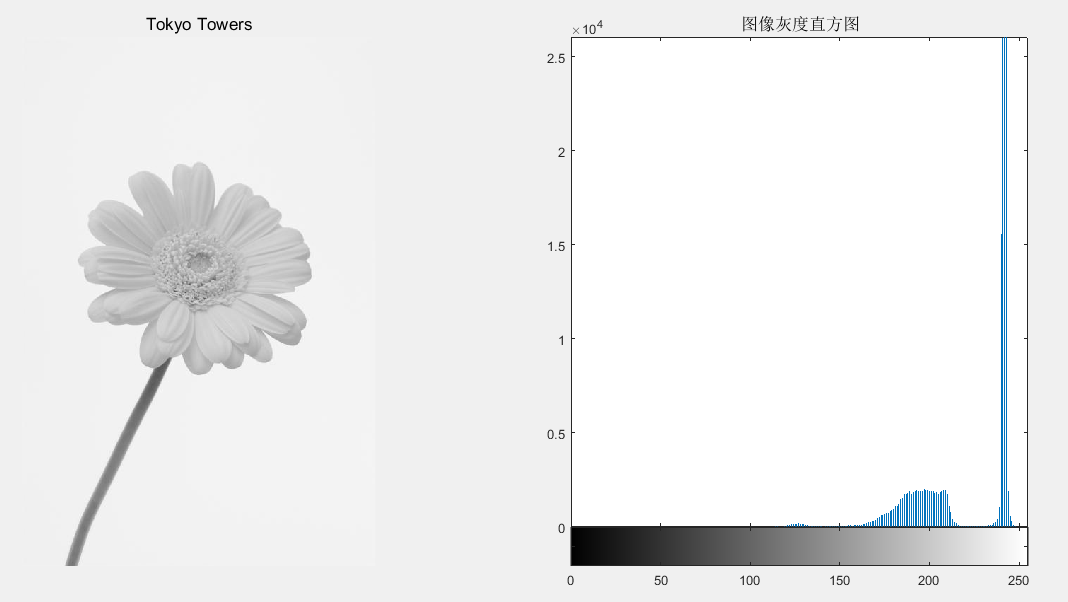## 5、图像分割（边缘提取）

### 1）图像几何纠正、边缘检测

B=imresize(A,m,type): 图像大小调整

B=imrotate(A,angle,method): 图像旋转调整

I=rgb2gray(InImg);
B1=imresize(I,1.5, 'nearest');
B2=imresize(I,1.5, 'bilinear');
C1= imrotate (I,45, 'nearest');
C2= imrotate (I,45, 'bilinear');
subplot(231);imshow(I) ;%显示原始图像于
title('原始图像');
subplot(232); imshow(B1) ;%显示调整图像(最近邻插值)
title('最近邻插值图像');
subplot(233); imshow(B2) ;% 显示调整图像(双线性插值)
title('双线性插值图像');
subplot(234); imshow(C1);% 显示旋转图像(最近邻插值)
title('旋转最近邻插值图像');
subplot(235); imshow(C2);% 显示旋转图像(双线性插值)
title('旋转双线性插值');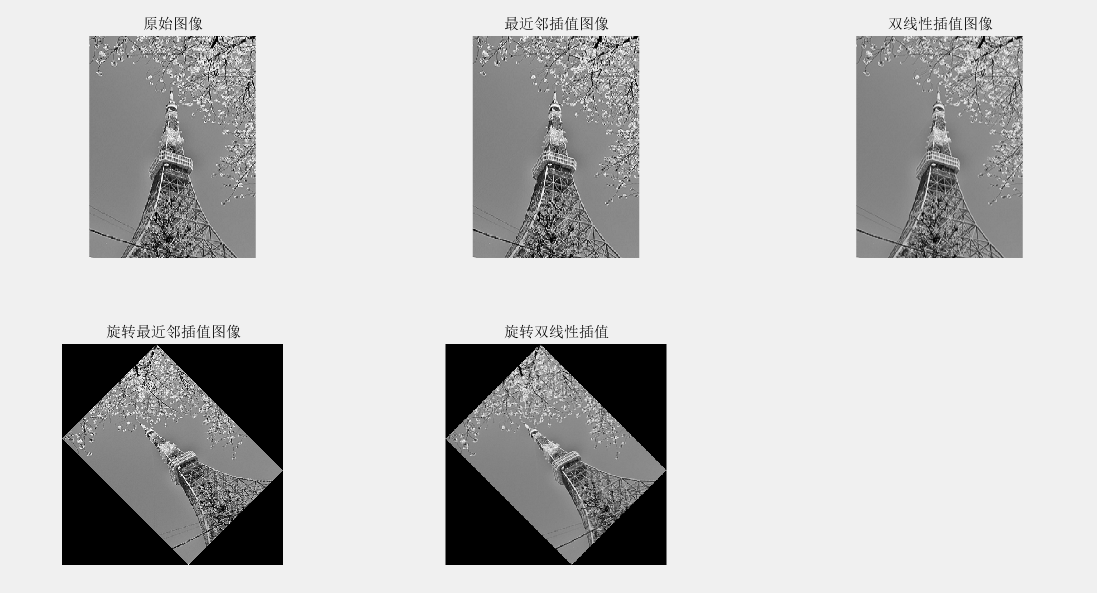2）边缘提取

BW=edge(I,method,thresh): 检测灰度图像的边缘

I=rgb2gray(InImg);
BW1= edge (I, 'sobel',0.1);%sobel边缘检测器
BW2= edge (I, 'prewitt',0.1);%prewitt边缘检测器
BW3= edge (I, 'roberts',0.1);%roberts边缘检测器
BW4= edge (I, 'log',0.01,2.0);%log边缘检测器
subplot(221); imshow(BW1) ;% 显示边缘图像
title('sobel边缘图像');
subplot(222); imshow(BW2) ;%显示边缘图像
title('prewitt边缘图像');
subplot(223); imshow(BW3) ;% 显示边缘图像
title('roberts边缘图像');
subplot(224); imshow(BW4) ;% 显示边缘图像
title('log边缘图像');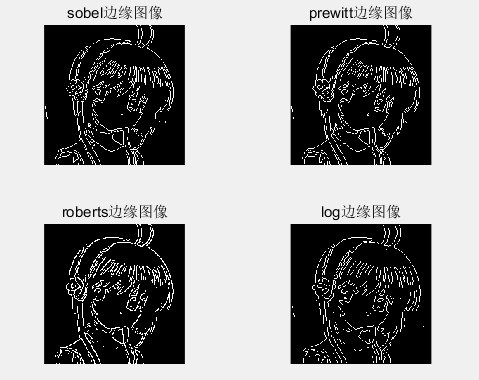wiener法图像处理 相关内容

## 数字图像处理，Matlab常用图像处理函数汇总

2016-03-03 14:39:15 EbowTang 阅读数 11534

# 图像的变换

j=fft2(i);

②ifft2:：ifft2函数用于数字图像的二维傅立叶反变换，如：

j=fft2(i);

k=ifft2(j);

# 噪声生成函数

① imnoise：用于对图像生成模拟噪声，如：

j=imnoise(i,'gaussian',0,0.02);%模拟高斯噪声

# 定义滤波器

② fspecial：用于产生预定义滤波器，如：

h=fspecial('sobel');%sobel水平边缘增强滤波器

h=fspecial('gaussian');%高斯低通滤波器

h=fspecial('laplacian');%拉普拉斯滤波器

h=fspecial('log');%高斯拉普拉斯（LoG）滤波器

h=fspecial('average');%均值滤波器

# 图像的增强

①直方图：imhist函数用于数字图像的直方图显示，如：

imhist(i);

②直方图均化：histeq函数用于数字图像的直方图均化，如：

j=histeq(i);

④对数变换：log函数用于数字图像的对数变换，如：

j=double(i);

k=log(j);

h=[1,2,1;0,0,0;-1,-2,-1];

j=filter2(h,i);

⑥线性滤波：利用二维卷积conv2滤波, 如:

h=[1,1,1;1,1,1;1,1,1];

h=h/9;

j=conv2(i,h);

⑦中值滤波：medfilt2函数用于图像的中值滤波，如：

j=medfilt2(i);

⑧锐化

（1）利用Sobel算子锐化图像, 如:

h=[1,2,1;0,0,0;-1,-2,-1];%Sobel算子

j=filter2(h,i);

（2）利用拉氏算子锐化图像, 如:

j=double(i);

h=[0,1,0;1,-4,0;0,1,0];%拉氏算子

k=conv2(j,h,'same');

m=j-k;

# 图像边缘检测

①sobel算子 如：

j = edge(i,'sobel',thresh)

②prewitt算子 如：

j = edge(i,'prewitt',thresh)

③roberts算子  如：

j = edge(i,'roberts',thresh)

④log算子  如：

j = edge(i,'log',thresh)

⑤canny算子 如：

j = edge(i,'canny',thresh)

⑥Zero-Cross算子 如：

j = edge(i,'zerocross',thresh)

# 形态学图像处理

①膨胀：是在二值化图像中“加长”或“变粗”的操作，函数imdilate执行膨胀运算，如：

b=[0 1 0;1 1 1;0 1 0];

c=imdilate(a,b);

②腐蚀：函数imerode执行腐蚀，如：

b=strel('disk',1);

c=imerode(a,b);

③开运算：先腐蚀后膨胀称为开运算，用imopen来实现，如：

b=strel('square',2);

c=imopen(a,b);

④闭运算：先膨胀后腐蚀称为闭运算，用imclose来实现，如：

b=strel('square',2);

c=imclose(a,b);

===========================================================

# Matlab 图像处理相关函数命令大全

## 一、通用函数：

colorbar  显示彩色条

getimage 从坐标轴取得图像数据

imshow 显示图像

montage 在矩形框中同时显示多幅图像

immovie 创建多帧索引图的电影动画

subimage 在一副图中显示多个图像

truesize 调整图像显示尺寸

warp 将图像显示到纹理映射表面

zoom 缩放图像

## 二、图像文件I/O函数命令

imfinfo  返回图形图像文件信息

imwrite  把图像写入（保存）图像文件中

imcrop  剪切图像

imresize  改变图像大小

imrotate  旋转图像

## 三、像素和统计处理函数

corr2  计算两个矩形的二维相关系数

imcontour 创建图像数据的轮廓图

imfeature  计算图像区域的特征尺寸

imbist  显示图像数据的柱状图

impixel 确定像素颜色值

[xi,yi,P]=impixel(x,y,...)

improfile 沿线段计算剖面图的像素值

mean2 计算矩阵元素的平均值

pixval  显示图像像素信息

std2 计算矩阵元素的标准偏移

## 四、图像分析函数：

edge 图像边缘检测

[BW,thresh]=edge(I,'prewitt',...)   BW=edge(I,'roberts') BW=edge(I,'roberts',thresh) [BW,thresh]=edge(I,'roberts',...) BW=edge(I,'log') BW=edge(I,'log',thresh) BW=edge(I,'log',thresh,sigma) [BW,threshold]=edge(I,'log',...) BW=edge(I,'zerocross',thresh,h) [BW,thresh]=edge(I,'zerocross',...)

BW=edge(I,'canny') BW=edge(I,'canny',thresh) BW=edge(I,'canny',thresh,sigma) [BW,threshold]=edge(I,'canny',...)

qtgetblk  获取四叉树分解的块值

qtsetblk 设置四叉树分解中的块值

## 五、图像增强函数

histeq 用柱状图均等化增强对比

imnoise 增强图像的渲染效果

medfilt2 进行二维中值过滤

ordfilt2 进行二维统计顺序过滤

wiener2 进行二维适应性去噪过滤处理

## 六、线性滤波函数

conv2 进行二维卷积操作

convmtx2 计算二维卷积矩阵

convn 计算n维卷积

filter2 进行二维线性过滤操作

fspecial 创建预定义过滤器

freqspace 确定二维频率响应的频率空间

freqz2 计算二维频率响应

fsamp2 用频率采样法设计二维FIR过滤器

ftrans2 通过频率转换设计二维FIR过滤器

fwind1 用一维窗口方法设计二维FIR过滤器

fwind2 用二维窗口方法设计二维FIR过滤器

## 八、图像变换函数

dct2 进行二维离散余弦变换(反余弦变换用idct2)

dctmtx 计算离散余弦傅立叶变换

fft2 进行二维快速傅立叶变换(反变换用ifft2)

fftn 进行n维快速傅立叶变换(反变换用ifftn)

fftshift 快速傅立叶变换的DC组件移到光谱中心

phantom 产生一个头部幻影图像

## 九、边沿和块处理函数

bestblk 确定进行块操作的块大小

blkproc 实现图像的显示块操作

col2im 将矩阵的列重新组织到块中

colfilt 利用列相关函数进行边沿操作

im2col 重调图像块为列

nlfilter 进行边沿操作

## 十、二进制图像操作函数

applylut 在二进制图像中利用lookup表进行行边沿操作

bwarea 计算二进制图像对象的面积

bweuler 计算二进制图像的欧拉数

bwfill 填充二进制图像的背景色

bwlabel 标注二进制图像中已连接的部分

bwmorph 提取二进制图像的轮廓

bwperim 计算二进制图像中对象的周长

bwselect 在二进制图像中选择对象

dilate 放大二进制图像

erode 弱化二进制图像的边界

makelut 创建一个用于applylut函数的lookup表

## 十一、区域处理函数

roicolor 选择感兴趣的颜色区

roifill 在图像的任意区域中进行平滑插补

roifilt2 过滤敏感区域

roipoly 选择一个敏感的多边形区域

## 十二、颜色映像处理函数

brighten 增加或降低颜色映像表的亮度

cmpermute 调整颜色映像表中的颜色

cmunigue 查找颜色映像表中特定的颜色及相应的图像

imapprox 对索引图像进行近似处理

rgbplot 划分颜色映像表

## 十三、颜色空间转换函数

hsv2rgb 转换HSV值为RGB颜色空间：M=hsv2rgb(H)

ntsc2rgb 转换NTSC值为RGB颜色空间：rgbmap=ntsc2rgb(yiqmap) RGB=ntsc2rgb(YIQ)

rgb2hsv 转换RGB值为HSV颜色空间：cmap=rgb2hsv(M)

rgb2ntsc 转换RGB值为NTSC颜色空间：yiqmap=rgb2ntsc(rgbmap) YIQ=rgb2ntsc(RGB)

rgb2ycbcr 转换RGB值为YCbCr颜色空间：ycbcrmap=rgb2ycbcr(rgbmap) YCBCR=rgb2ycbcr(RGB)

ycbcr2rgb 转化YCbCr值为RGB颜色空间：rgbmap=ycbcr2rgb(ycbcrmap) RGB=ycbcr2rgb(YCBCR)

## 十四、图像类型和类型转换函数

dither 通过抖动增加外观颜色分辨率转换图像

gray2ind 转换灰度图像为索引图像

grayslice 从灰度图像为索引图像

im2bw 转换图像为二进制图像

im2double 转换图像矩阵为双精度型

double 转换数据为双精度型

unit8 、unit16转换数据为8位、16位无符号整型： i=unit8(x) i=unit16(x)

im2unit8 转换图像阵列为8位无符号整型

im2unit16 转换图像阵列为16位无符号整型

ind2gray 把检索图像转化为灰度图像

ind2rgb  转化索引图像为RGB真彩图像

isbw 判断是否为二进制图像

isgray 判断是否为灰度图像

isind 判断是否为索引图像

isrgb 判断是否为RGB真彩色图像

mat2gray 转换矩阵为灰度图像

rgb2gray 转换RGB图像或颜色映像表为灰度图像

rgb2ind 转换RGB图像为索引图像

## 十五、新增图像处理工具箱函数

applycform 用于颜色空间变换 out=applyform(I,C)

bwboundaries 描绘二进制图像边界

bwtraceboundary 描述二进制图像中的物体

B=bwtraceboundary(BW,P,fstep) B=bwtraceboundary(BW,P,fstep,CONN) B=bwtraceboundary(...N,dir)

decorrstrech 对多通道图像进行去相关处理

dicomdict 获取或读取DICOM文件

getline 用鼠标选择ployline

getpts 用鼠标选择像素点

getrect 用鼠标选择矩阵

im2java2d 将图像转换为Java缓冲图像

imview 在图像与蓝旗中显示图像

ippl 检查IPPL的存在

iptdemos 显示图像处理工具箱中的索引图像

lab2double、lab2unit16、lab2unit8 将L*a*b数据分别转换为双精度、16位数据、8位数据

makecform 创造一个色彩转换结构

unitlut 查找表中A像素值

xyz2double、xyz2unit16 将颜色数据从XYZ转换到双精度、16进制。

wiener法图像处理 相关内容

## matlab图像处理命令(二)

2010-08-20 14:38:00 fengbingchun 阅读数 9822

1. 直方图均衡化的 Matlab 实现
1.1 imhist 函数

imhist(X,map)

stem(x,counts) 同样可以显示直方图。
1.2 imcontour 函数

[bottom top] 都为2×3的矩阵，则分别调整 R、G、B 3个分量。
1.4 histeq 函数

J=histeq(I,n)
[J,T]=histeq(I,...)
newmap=histeq(X,map,hgram)
newmap=histeq(X,map)
[new,T]=histeq(X,...)

2. 噪声及其噪声的 Matlab 实现
imnoise 函数

J=imnoise(I,type,parameter)

3. 图像滤波的 Matlab 实现
3.1 conv2 函数

C=conv2(Hcol,Hrow,A)
C=conv2(...,'shape')

C=conv2(Hcol,Hrow,A) 中，矩阵 A 分别与 Hcol 向量在列方向和 Hrow 向量在行方向上进行卷积；C=conv2(...,'shape') 用来指定 conv2

》full 为缺省值，返回二维卷积的全部结果；
》same 返回二维卷积结果中与 A 大小相同的中间部分；
valid 返回在卷积过程中，未使用边缘补 0 部分进行计算的卷积结果部分，当 size(A)>size(B) 时，size(C)=[Ma-Mb+1,Na-Nb+1]

3.2 conv 函数

3.3 filter2函数

Y=filter2(B,X,'shape')

》full 返回二维相关的全部结果，size(Y)>size(X)；
》same 返回二维互相关结果的中间部分，Y 与 X 大小相同；
》valid 返回在二维互相关过程中，未使用边缘补 0 部分进行计算的结果部分，有 size(Y)<size(X) 。
3.4 fspecial 函数

H=fspecial('gaussian',n,sigma)         高斯低通滤波器
H=fspecial('sobel')                          Sobel 水平边缘增强滤波器
H=fspecial('prewitt')                       Prewitt 水平边缘增强滤波器
H=fspecial('laplacian',alpha)             近似二维拉普拉斯运算滤波器
H=fspecial('log',n,sigma)                 高斯拉普拉斯（LoG）运算滤波器
H=fspecial('average',n)                   均值滤波器
H=fspecial('unsharp',alpha)             模糊对比增强滤波器

4. 彩色增强的 Matlab 实现
4.1 imfilter函数

1. 离散傅立叶变换的 Matlab 实现
Matlab 函数 fft、fft2 和 fftn 分别可以实现一维、二维和 N 维 DFT 算法；而函数 ifft、ifft2 和 ifftn 则用来计算反 DFT 。

A＝fft(X,N,DIM)
其中，X 表示输入图像；N 表示采样间隔点，如果 X 小于该数值，那么 Matlab 将会对 X 进行零填充，否则将进行截取，使之长度为

N ；DIM 表示要进行离散傅立叶变换。
A＝fft2(X,MROWS,NCOLS)

A＝fftn(X,SIZE)

函数 ifft、ifft2 和 ifftn的调用格式于对应的离散傅立叶变换函数一致。

% 读入原始图像
imshow(I)
% 求离散傅立叶频谱
J=fftshift(fft2(I));
figure;
imshow(log(abs(J)),[8,10])
2. 离散余弦变换的 Matlab 实现
2.1. dCT2 函数

B=dct2(A,m,n)
B=dct2(A,[m,n])

2.2. dict2 函数

B=idct2(A,m,n)
B=idct2(A,[m,n])

2.3. dctmtx函数

3. 图像小波变换的 Matlab 实现
3.1 一维小波变换的 Matlab 实现
(1) dwt 函数

[cA,cD]=dwt(X,Lo_D,Hi_D)

(2) idwt 函数

X=idwt(cA,cD,Lo_R,Hi_R)
X=idwt(cA,cD,'wname',L)
X=idwt(cA,cD,Lo_R,Hi_R,L)

'wname' 为所选的小波函数
X=idwt(cA,cD,Lo_R,Hi_R) 用指定的重构滤波器 Lo_R 和 Hi_R 经小波反变换重构原始信号 X 。
X=idwt(cA,cD,'wname',L) 和 X=idwt(cA,cD,Lo_R,Hi_R,L) 指定返回信号 X 中心附近的 L 个点。
3.2 二维小波变换的 Matlab 实现
二维小波变换的函数
-------------------------------------------------
函数名                函数功能
---------------------------------------------------
dwt2            二维离散小波变换
wavedec2       二维信号的多层小波分解
idwt2           二维离散小波反变换
waverec2        二维信号的多层小波重构
wrcoef2          由多层小波分解重构某一层的分解信号
upcoef2          由多层小波分解重构近似分量或细节分量
detcoef2         提取二维信号小波分解的细节分量
appcoef2        提取二维信号小波分解的近似分量
upwlev2         二维小波分解的单层重构
dwtpet2         二维周期小波变换
idwtper2        二维周期小波反变换
-------------------------------------------------------------
(1) wcodemat 函数

Y=wcodemat(X,NB,OPT)
Y=wcodemat(X,NB)
Y=wcodemat(X)

OPT 指定了编码的方式（缺省值为 'mat'），即：
OPT＝'row' ，按行编码
OPT＝'col' ，按列编码
OPT＝'mat' ，按整个矩阵编码
ABSOL 是函数的控制参数（缺省值为 '1'），即：
ABSOL＝0 时，返回编码矩阵
ABSOL＝1 时，返回数据矩阵的绝对值 ABS(X)
(2) dwt2 函数

[cA,cH,cV,cD]=dwt2(X,Lo_D,Hi_D)

(3) wavedec2 函数

[C,S]=wavedec2(X,N,Lo_D,Hi_D)

(4) idwt2 函数

X=idwt2(cA,cH,cV,cD,Lo_R,Hi_R)
X=idwt2(cA,cH,cV,cD,'wname',S)
X=idwt2(cA,cH,cV,cD,Lo_R,Hi_R,S)

；X=idwt2(cA,cH,cV,cD,Lo_R,Hi_R) 使用指定的重构低通和高通滤波器 Lo_R 和 Hi_R 重构原信号 X ；X=idwt2(cA,cH,cV,cD,'wname',S)

(5) waverec2 函数

X=waverec2(C,S,Lo_R,Hi_R)

1. 图像和图像数据
缺省情况下，MATLAB将图像中的数据存储为双精度类型(double)，64位浮点

在使用MATLAB工具箱时，一定要注意函数所要求的参数类型。另外，uint8

从uint8到double的转换
---------------------------------------------
图像类型        MATLAB语句
---------------------------------------------
索引色             B=double(A)+1
索引色或真彩色 B=double(A)/255
二值图像          B=double(A)
---------------------------------------------
从double到uint8的转换
---------------------------------------------
图像类型        MATLAB语句
---------------------------------------------
索引色               B=uint8(round(A-1))
索引色或真彩色    B=uint8(round(A*255))
二值图像            B=logical(uint8(round(A)))
---------------------------------------------
2. 图像处理工具箱所支持的图像类型
2.1 真彩色图像
R、G、B三个分量表示一个像素的颜色。如果要读取图像中(100,50)处的像素值，

真彩色图像可用双精度存储，亮度值范围是[0,1]；比较符合习惯的存储方法是用无

2.2 索引色图像
包含两个结构，一个是调色板，另一个是图像数据矩阵。调色板是一个有3列和若干行

注意：MATLAB中调色板色彩强度[0,1]，0代表最暗，1代表最亮。
常用颜色的RGB值
--------------------------------------------
颜色    R   G   B      颜色    R   G   B
--------------------------------------------
黑     0   0   1      洋红    1   0   1
白     1   1   1      青蓝    0   1   1
红     1   0   0      天蓝 0.67 0   1
绿     0   1   0      橘黄    1 0.5 0
蓝     0   0   1      深红   0.5 0   0
黄     1   1   0       灰    0.5 0.5 0.5
--------------------------------------------
产生标准调色板的函数
-------------------------------------------------
函数名       调色板
-------------------------------------------------
Hsv       色彩饱和度，以红色开始，并以红色结束
Hot       黑色－红色－黄色－白色
Cool      青蓝和洋红的色度
Pink      粉红的色度
Gray      线型灰度
Bone      带蓝色的灰度
Jet        Hsv的一种变形，以蓝色开始，以蓝色结束
Copper    线型铜色度
Prim       三棱镜，交替为红、橘黄、黄、绿和天蓝
Flag       交替为红、白、蓝和黑
--------------------------------------------------
缺省情况下，调用上述函数灰产生一个64×3的调色板，用户也可指定调色板大小。
索引色图像数据也有double和uint8两种类型。
当图像数据为double类型时，值1代表调色板中的第1行，值2代表第2行……
如果图像数据为uint8类型，0代表调色板的第一行，，值1代表第2行……
2.3 灰度图像
存储灰度图像只需要一个数据矩阵。
数据类型可以是double，[0，1]；也可以是uint8，[0,255]
2.4 二值图像
二值图像只需一个数据矩阵，每个像素只有两个灰度值，可以采用uint8或double类型存储。
MATLAB工具箱中以二值图像作为返回结果的函数都使用uint8类型。
2.5 图像序列
MATLAB工具箱支持将多帧图像连接成图像序列。
图像序列是一个4维数组，图像帧的序号在图像的长、宽、颜色深度之后构成第4维。
分散的图像也可以合并成图像序列，前提是各图像尺寸必须相同，若是索引色图像，

可参考cat()函数    A＝cat(4,A1,A2,A3,A4,A5)
3. MATLAB图像类型转换
图像类型转换函数
---------------------------------------------------------------------------
函数名                      函数功能
---------------------------------------------------------------------------
dither       图像抖动，将灰度图变成二值图，或将真彩色图像抖动成索引色图像
gray2ind    将灰度图像转换成索引图像
grayslice    通过设定阈值将灰度图像转换成索引色图像
im2bw      通过设定亮度阈值将真彩色、索引色、灰度图转换成二值图
ind2gray    将索引色图像转换成灰度图像
ind2rgb      将索引色图像转换成真彩色图像
mat2gray   将一个数据矩阵转换成一副灰度图
rgb2gray    将一副真彩色图像转换成灰度图像
rgb2ind      将真彩色图像转换成索引色图像
----------------------------------------------------------------------------
4. 图像文件的读写和查询
4.1 图形图像文件的读取
通常，读取的大多数图像均为8bit，当这些图像加载到内存中时，Matlab就将其存放

4.2 图形图像文件的写入
使用imwrite函数，语法如下：
imwrite(A,filename,fmt)
imwrite(X,map,filename,fmt)
imwrite(...,filename)
imwrite(...,parameter,value)
当利用imwrite函数保存图像时，Matlab缺省的方式是将其简化道uint8的数据格式。
4.3 图形图像文件信息的查询   imfinfo()函数
5. 图像文件的显示
5.1 索引图像及其显示
方法一：
image(X)
colormap(map)
方法二：
imshow(X,map)
5.2 灰度图像及其显示
Matlab 7.0 中，要显示一副灰度图像，可以调用函数 imshow 或 imagesc （即
imagescale，图像缩放函数）
(1) imshow 函数显示灰度图像
使用 imshow(I)    或使用明确指定的灰度级书目：imshow(I,32)
由于Matlab自动对灰度图像进行标度以适合调色板的范围，因而可以使用自定义

imshow(I,[low,high])
其中，low 和 high 分别为数据数组的最小值和最大值。
(2) imagesc 函数显示灰度图像
下面的代码是具有两个输入参数的 imagesc 函数显示一副灰度图像
imagesc(1,[0,1]);
colormap(gray);
imagesc 函数中的第二个参数确定灰度范围。灰度范围中的第一个值（通常是0），

在调用 imagesc 函数时，若只使用一个参数，可以用任意灰度范围显示图像。在该

5.3 RGB 图像及其显示
(1) image(RGB)
不管RGB图像的类型是double浮点型，还是 uint8 或 uint16 无符号整数型，Matlab都

RGB8 = uint8(round(RGB64×255)); ％ 将 double 浮点型转换为 uint8 无符号整型
RGB64 = double(RGB8)/255;            ％ 将 uint8 无符号整型转换为 double 浮点型
RGB16 = uint16(round(RGB64×65535)); ％ 将 double 浮点型转换为 uint16 无符号整型
RGB64 = double(RGB16)/65535;      ％ 将 uint16 无符号整型转换为 double 浮点型
(2) imshow(RGB) 参数是一个 m×n×3 的数组
5.4 二进制图像及其显示
(1) imshow(BW)
在 Matlab 7.0 中，二进制图像是一个逻辑类，仅包括 0 和 1 两个数值。像素 0 显示

显示时，也可通过NOT(~)命令，对二进制图象进行取反，使数值 0 显示为白色；1 显示

例如： imshow(~BW)
(2) 此外，还可以使用一个调色板显示一副二进制图像。如果图形是 uint8 数据类型，

例如： imshow(BW,[1 0 0;0 0 1])
5.5 直接从磁盘显示图像
可使用一下命令直接进行图像文件的显示：
imshow filename
其中，filename 为要显示的图像文件的文件名。
如果图像是多帧的，那么 imshow 将仅显示第一帧。但需注意，在使用这种方式时，图像

命令形式为： rgb ＝ getimage;

bwlabel

L = bwlabel(BW,n)
[L,num] = bwlabel(BW,n)

isbw

flag = isbw(A)

isind, isgray, isrgb
74．isgray

flag = isgray(A)

isbw, isind, isrgb

11．bwselect

BW2 = bwselect(BW1,c,r,n)
BW2 = bwselect(BW1,n)
[BW2,idx] = bwselect(...)

c = [16 90 144];
r = [85 197 247];
BW2 = bwselect(BW1,c,r,4);
imshow(BW1)
figure, imshow(BW2)

47．im2bw

BW = im2bw(I,level)
BW = im2bw(X,map,level)
BW = im2bw(RGB,level)

BW = im2bw(X,map,0.4);
imshow(X,map)

1、图像的变换

j=fft2(i);

②ifft2:：ifft2函数用于数字图像的二维傅立叶反变换，如：

j=fft2(i);

k=ifft2(j);

2、模拟噪声生成函数和预定义滤波器

① imnoise：用于对图像生成模拟噪声，如：

j=imnoise(i,'gaussian',0,0.02);%模拟高斯噪声

② fspecial：用于产生预定义滤波器，如：

h=fspecial('sobel');%sobel水平边缘增强滤波器

h=fspecial('gaussian');%高斯低通滤波器

h=fspecial('laplacian');%拉普拉斯滤波器

h=fspecial('log');%高斯拉普拉斯（LoG）滤波器

h=fspecial('average');%均值滤波器

2、图像的增强

①直方图：imhist函数用于数字图像的直方图显示，如：

imhist(i);

②直方图均化：histeq函数用于数字图像的直方图均化，如：

j=histeq(i);

④对数变换：log函数用于数字图像的对数变换，如：

j=double(i);

k=log(j);

h=[1,2,1;0,0,0;-1,-2,-1];

j=filter2(h,i);

⑥线性滤波：利用二维卷积conv2滤波, 如:

h=[1,1,1;1,1,1;1,1,1];

h=h/9;

j=conv2(i,h);

⑦中值滤波：medfilt2函数用于图像的中值滤波，如：

j=medfilt2(i);

⑧锐化

（1）利用Sobel算子锐化图像, 如:

h=[1,2,1;0,0,0;-1,-2,-1];%Sobel算子

j=filter2(h,i);

（2）利用拉氏算子锐化图像, 如:

j=double(i);

h=[0,1,0;1,-4,0;0,1,0];%拉氏算子

k=conv2(j,h,'same');

m=j-k;

3、图像边缘检测

①sobel算子 如：

j = edge(i,'sobel',thresh)

②prewitt算子 如：

j = edge(i,'prewitt',thresh)

③roberts算子  如：

j = edge(i,'roberts',thresh)

④log算子  如：

j = edge(i,'log',thresh)

⑤canny算子 如：

j = edge(i,'canny',thresh)

⑥Zero-Cross算子 如：

j = edge(i,'zerocross',thresh)

4、形态学图像处理

①膨胀：是在二值化图像中“加长”或“变粗”的操作，函数imdilate执行膨胀运算，如：

b=[0 1 0;1 1 1;0 1 0];

c=imdilate(a,b);

②腐蚀：函数imerode执行腐蚀，如：

b=strel('disk',1);

c=imerode(a,b);

③开运算：先腐蚀后膨胀称为开运算，用imopen来实现，如：

b=strel('square',2);

c=imopen(a,b);

④闭运算：先膨胀后腐蚀称为闭运算，用imclose来实现，如：

b=strel('square',2);

c=imclose(a,b);

===========================================================

Matlab 图像处理相关函数命令大全

一、通用函数：

colorbar  显示彩色条

getimage 从坐标轴取得图像数据

imshow 显示图像

montage 在矩形框中同时显示多幅图像

immovie 创建多帧索引图的电影动画

subimage 在一副图中显示多个图像

truesize 调整图像显示尺寸

warp 将图像显示到纹理映射表面

zoom 缩放图像

二、图像文件I/O函数命令

imfinfo  返回图形图像文件信息

imwrite  把图像写入（保存）图像文件中

imcrop  剪切图像

imresize  改变图像大小

imrotate  旋转图像

三、像素和统计处理函数

corr2  计算两个矩形的二维相关系数

imcontour 创建图像数据的轮廓图

imfeature  计算图像区域的特征尺寸

imbist  显示图像数据的柱状图

impixel 确定像素颜色值

[xi,yi,P]=impixel(x,y,...)
improfile 沿线段计算剖面图的像素值

mean2 计算矩阵元素的平均值

pixval  显示图像像素信息

std2 计算矩阵元素的标准偏移

edge 图像边缘检测

[BW,thresh]=edge(I,'prewitt',...) \  BW=edge(I,'roberts') \ BW=edge(I,'roberts',thresh) \ [BW,thresh]=edge(I,'roberts',...) \ BW=edge(I,'log') \ BW=edge(I,'log',thresh) \ BW=edge(I,'log',thresh,sigma) \ [BW,threshold]=edge(I,'log',...) \ BW=edge(I,'zerocross',thresh,h) \ [BW,thresh]=edge(I,'zerocross',...) \

BW=edge(I,'canny') \ BW=edge(I,'canny',thresh) \ BW=edge(I,'canny',thresh,sigma) \ [BW,threshold]=edge(I,'canny',...)

qtgetblk  获取四叉树分解的块值

qtsetblk 设置四叉树分解中的块值

histeq 用柱状图均等化增强对比

imnoise 增强图像的渲染效果

medfilt2 进行二维中值过滤

ordfilt2 进行二维统计顺序过滤

wiener2 进行二维适应性去噪过滤处理

conv2 进行二维卷积操作

convmtx2 计算二维卷积矩阵

convn 计算n维卷积

filter2 进行二维线性过滤操作

fspecial 创建预定义过滤器

freqspace 确定二维频率响应的频率空间

freqz2 计算二维频率响应

fsamp2 用频率采样法设计二维FIR过滤器

ftrans2 通过频率转换设计二维FIR过滤器

fwind1 用一维窗口方法设计二维FIR过滤器

fwind2 用二维窗口方法设计二维FIR过滤器

dct2 进行二维离散余弦变换(反余弦变换用idct2)

dctmtx 计算离散余弦傅立叶变换

fft2 进行二维快速傅立叶变换(反变换用ifft2)

fftn 进行n维快速傅立叶变换(反变换用ifftn)

fftshift 快速傅立叶变换的DC组件移到光谱中心

phantom 产生一个头部幻影图像

bestblk 确定进行块操作的块大小

blkproc 实现图像的显示块操作

col2im 将矩阵的列重新组织到块中

colfilt 利用列相关函数进行边沿操作

im2col 重调图像块为列

nlfilter 进行边沿操作

applylut 在二进制图像中利用lookup表进行行边沿操作

bwarea 计算二进制图像对象的面积

bweuler 计算二进制图像的欧拉数

bwfill 填充二进制图像的背景色

bwlabel 标注二进制图像中已连接的部分

bwmorph 提取二进制图像的轮廓

bwperim 计算二进制图像中对象的周长

bwselect 在二进制图像中选择对象

dilate 放大二进制图像

erode 弱化二进制图像的边界

makelut 创建一个用于applylut函数的lookup表

roicolor 选择感兴趣的颜色区

roifill 在图像的任意区域中进行平滑插补

roifilt2 过滤敏感区域

roipoly 选择一个敏感的多边形区域

brighten 增加或降低颜色映像表的亮度

cmpermute 调整颜色映像表中的颜色

cmunigue 查找颜色映像表中特定的颜色及相应的图像

imapprox 对索引图像进行近似处理

rgbplot 划分颜色映像表

hsv2rgb 转换HSV值为RGB颜色空间：M=hsv2rgb(H)

ntsc2rgb 转换NTSC值为RGB颜色空间：rgbmap=ntsc2rgb(yiqmap) \ RGB=ntsc2rgb(YIQ)

rgb2hsv 转换RGB值为HSV颜色空间：cmap=rgb2hsv(M)

rgb2ntsc 转换RGB值为NTSC颜色空间：yiqmap=rgb2ntsc(rgbmap) \ YIQ=rgb2ntsc(RGB)

rgb2ycbcr 转换RGB值为YCbCr颜色空间：ycbcrmap=rgb2ycbcr(rgbmap) \ YCBCR=rgb2ycbcr(RGB)

ycbcr2rgb 转化YCbCr值为RGB颜色空间：rgbmap=ycbcr2rgb(ycbcrmap) \ RGB=ycbcr2rgb(YCBCR)

dither 通过抖动增加外观颜色分辨率转换图像

gray2ind 转换灰度图像为索引图像

grayslice 从灰度图像为索引图像

im2bw 转换图像为二进制图像

im2double 转换图像矩阵为双精度型

double 转换数据为双精度型

unit8 、unit16转换数据为8位、16位无符号整型： i=unit8(x) \ i=unit16(x)

im2unit8 转换图像阵列为8位无符号整型

im2unit16 转换图像阵列为16位无符号整型

ind2gray 把检索图像转化为灰度图像

ind2rgb  转化索引图像为RGB真彩图像

isbw 判断是否为二进制图像

isgray 判断是否为灰度图像

isind 判断是否为索引图像

isrgb 判断是否为RGB真彩色图像

mat2gray 转换矩阵为灰度图像

rgb2gray 转换RGB图像或颜色映像表为灰度图像

rgb2ind 转换RGB图像为索引图像

applycform 用于颜色空间变换 out=applyform(I,C)

bwboundaries 描绘二进制图像边界

bwtraceboundary 描述二进制图像中的物体

B=bwtraceboundary(BW,P,fstep) \ B=bwtraceboundary(BW,P,fstep,CONN) \ B=bwtraceboundary(...N,dir)

decorrstrech 对多通道图像进行去相关处理

dicomdict 获取或读取DICOM文件

getline 用鼠标选择ployline

getpts 用鼠标选择像素点

getrect 用鼠标选择矩阵

im2java2d 将图像转换为Java缓冲图像

imview 在图像与蓝旗中显示图像

ippl 检查IPPL的存在

iptdemos 显示图像处理工具箱中的索引图像

lab2double、lab2unit16、lab2unit8 将L*a*b数据分别转换为双精度、16位数据、8位数据

makecform 创造一个色彩转换结构

unitlut 查找表中A像素值

xyz2double、xyz2unit16 将颜色数据从XYZ转换到双精度、16进制。

wiener法图像处理 相关内容